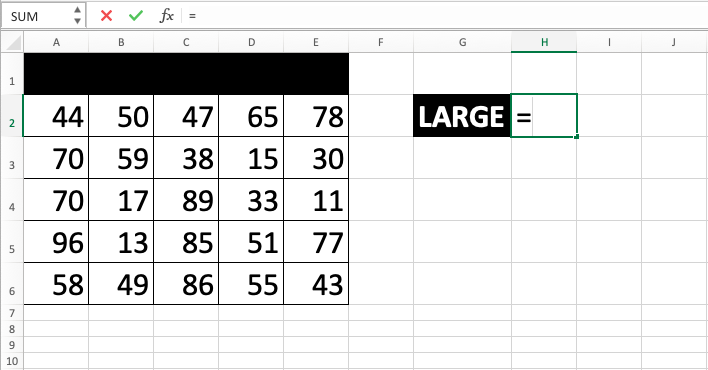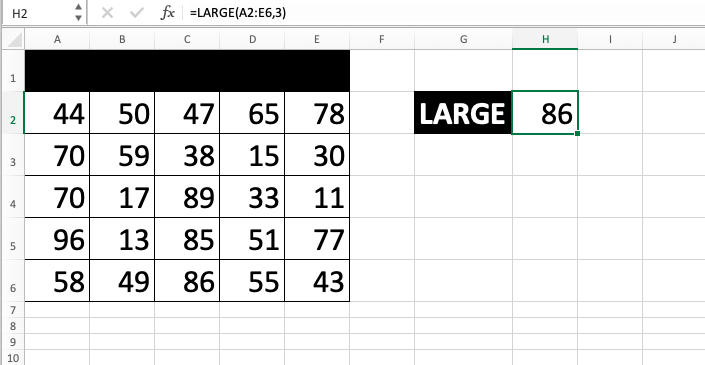LARGE Function in Excel - Compute Expert

# LARGE Function in Excel

In this tutorial, you will learn the way to use excel LARGE formula. LARGE function in excel can be used to find a number based on its descending rank in a cell range on a spreadsheet.

## Why We Need to Learn About Excel LARGE Formula?

In processing numeral data on a spreadsheet, often we need to know which number has the nth largest value compared to other numeral data. This is sometimes needed to do further analysis and/or so we understand the ranking in a group of digits that we have in the spreadsheet, which can also be the base of some particular indicators.

There are many examples where we need the knowledge on the rank of numbers that we have based on the largeness of the value. Things like product sales, employee performance, production target, revenue and spending, vendor determination in procurement, and others often need us to know numbers based on their descending rank as the base of company policy-making for related division and also as a way to check the performance of the company in various important aspects that we need to look into.

The info of number based on the nth largest rank compared to other value is also needed for further data analysis process material. For example, if we know which company product has the largest sales in a period, then we can analyze the other data of the product. What makes it getting the largest sales number? How can the other products show similar performance? As shown, this can be the base of other important analysis for the company.

If there are many digits to check based on their nth largest rank, then it will be difficult to do it manually.

For that matter, you can use LARGE function in excel. Excel LARGE formula can help to find a number based on its nth largest rank in a particular cell range. Excel LARGE formula is very useful to be learned and understood if you often play with various kinds of numeral data on a spreadsheet.

## What is LARGE Function in Excel?

LARGE function in excel is a function with the usage objective to get a number based on its nth largest rank in a cell range on a spreadsheet. Excel LARGE formula has its formula pair with the opposite function, to get a number based on its nth smallest rank, which is SMALL. The way to use them is similar to one another.

Here is a little bit of explanation about the inputs in the formula:

=LARGE(array, k)

Note:
• array = cell range where the numbers that you want to find the k-th largest value are
• k = the k-th largest value that you want to find from your group of numbers

## How to Use Excel LARGE Formula?

The following will explain how to use LARGE function in excel. Excel LARGE formula needs inputs of a cell range contains all digits for the descending rank comparison and the rank of the number that we want to find based on the largeness of its value compared to all digits in the previously inputted cell range.

## Using Excel LARGE Function

1. Type equal sign ( = ) in the cell where you want to put the result in2. Type LARGE (can be with large and small letters) and open bracket sign after =3. Drag cursor from the most top left to the most bottom right on the cell range containing all the numbers that you want to compare the descending order rank at. After that, type comma sign ( , )4. Type rank number/cell coordinate where the nth largest rank number that you want to find the number compared to the other digits value in the previous cell range is5. Type close bracket sign6. Press Enter
7. The process is done!## Exercise

After you have learned how to use the excel LARGE formula above, you can practice your understanding by doing the exercise below!

### Questions

1. What is the highest mathematic score test? Fill the answer in the provided cell and mark the name of the student who has the score with red color!
2. What is the third-highest language score test? Fill the answer in the provided cell and mark the name of the student who has the score with yellow color!
3. What is the fifth-highest physics score test? Fill the answer in the provided cell and mark the name of the student who has the score with green color!

• If you want to get a digit based on the nth smallest rank, then you can use SMALL formula
• If you want to get the rank not the digit, then you can use RANK formula

Get updated excel info from Compute Expert by registering your email. It's free!